# Maharashtra Board Class 9 Maths Solutions Chapter 8 Trigonometry Practice Set 8.1

## Maharashtra State Board Class 9 Maths Solutions Chapter 8 Trigonometry Practice Set 8.1

Question 1.
In the given figure, ∠R is the right angle of ∆PQR. Write the following ratios.i. sin P
ii. cos Q
iii. tan P
iv. tan Q
Solution: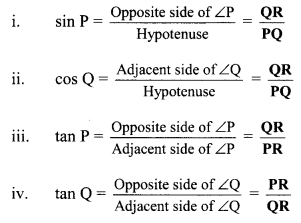Question 2.
In the right angled ∆XYZ, ∠XYZ = 90° and a, b, c are the lengths of the sides as shown in the figure. Write the following ratios.i. sin x
ii. tan z
iii. cos x
iv. tan x.
Solution: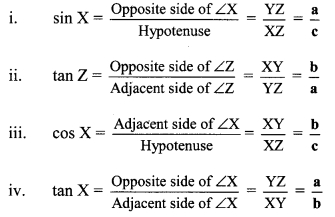Question 3.
In right angled ∆LMN, ∠LMN = 90°, ∠L = 50° and ∠N = 40°. Write the following ratios.i. sin 50°
ii. cos 50°
iii. tan 40°
iv. cos 40°
Solution:Question 4.
In the given figure, ∠PQR = 90°, ∠PQS = 90°, ∠PRQ = α and ∠QPS = θ. Write the following trigonometric ratios.
i. sin α, cos α , tan α
ii. sin θ, cos θ, tan θSolution:
i. In ∆PQR,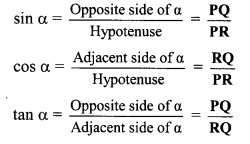ii. In ∆PQS,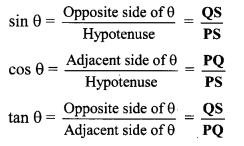Maharashtra Board Class 9 Maths Chapter 8 Trigonometry Practice Set 8.1 Intext Questions and Activities

Question 1.
In the figure gIven below, ∆PQR is a right angled triangle. Write the names of sides opposite and adjacent to ∠P and ∠R. (Textbook pg no. 102)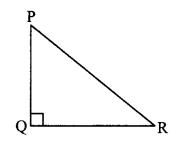Solution:
In right angled ∆PQR,
i. side opposite to ∠P = QR
ii. side opposite to ∠R = PQ
iii. side adjacent to ∠P = PQ
iv. side adjacent to ∠R = QR